Cui, H., Li, T., Chen, T., Wu, L., Li, H., Liu, C., Xin, M., and Song, Y. (2023). "Parameter calibration for simulation of Gentiana seedling substrate based on discrete element method," BioResources 18(1), 1999-2010.

#### Abstract

The physical and mechanical parameters of the rotten rice straw (RRS), rice husk biochar (RHB), and the mixture of the two materials (the substrate) were calibrated by Plackett-Burman and Box-Behnken experiments to obtain the parameters for simulation of the forming of the Gentiana seedling substrate mat (GSSM). The particle contact parameters were calibrated, and the repose angle was taken as the response value based on the Hertz-Mindlin approach with the JKR contact model of discrete element method (DEM). A quadratic regression model was established and optimized using Design-Expert software. The parameters that most affected the substrate repose angle were the restitution coefficient of RRS of 0.20, the rolling friction coefficient of RRS of 0.04, the surface energy of RRS for JKR of 0.53, and the surface energy of RHB-RRS for JKR of 2.11. The simulated repose angle of the substrate and the bending strength of GSSM were compared with that of the verified experimental values respectively based on the optimal parameters. The relative errors of repose angles and bending strengths between the values of the simulation and the measurement were 0.71% and 1.39% respectively, indicating that the parameters obtained in this study can provide a reliable reference for the forming of GSSM.

Parameter Calibration for Simulation of Gentiana Seedling Substrate Based on Discrete Element Method

Hongguang Cui,a Tian Li,a Tianyou Chen,a Liyan Wu,a Hongbo Li,b Cuihong Liu,a Mingjin Xin,a and Yuqiu Song a,*

The physical and mechanical parameters of the rotten rice straw (RRS), rice husk biochar (RHB), and the mixture of the two materials (the substrate) were calibrated by Plackett-Burman and Box-Behnken experiments to obtain the parameters for simulation of the forming of the Gentiana seedling substrate mat (GSSM). The particle contact parameters were calibrated, and the repose angle was taken as the response value based on the Hertz-Mindlin approach with the JKR contact model of discrete element method (DEM). A quadratic regression model was established and optimized using Design-Expert software. The parameters that most affected the substrate repose angle were the restitution coefficient of RRS of 0.20, the rolling friction coefficient of RRS of 0.04, the surface energy of RRS for JKR of 0.53, and the surface energy of RHB-RRS for JKR of 2.11. The simulated repose angle of the substrate and the bending strength of GSSM were compared with that of the verified experimental values respectively based on the optimal parameters. The relative errors of repose angles and bending strengths between the values of the simulation and the measurement were 0.71% and 1.39% respectively, indicating that the parameters obtained in this study can provide a reliable reference for the forming of GSSM.

DOI: 10.15376/biores.18.1.1999-2010

Keywords: Rotten rice straw; Biochar; Repose angle; Parameter calibration; Gentiana seedling substrate mat

Contact information: a: College of Engineering, Shenyang Agricultural University, Shenyang 110866 China; b: College of Horticultural, Shenyang Agricultural University, Shenyang 110866 China;

* Corresponding author: songyuqiu@syau.edu.cn

INTRODUCTION

The genus Gentiana is the source of a Chinese herbal medicine that has been applied for medicinal uses such as anti-rheumatic, anti-inflammatory, analgesic, antipyretic, hypoglycemic, and diuretic purposes (Rodrigues et al. 2019). However, the germination rate of traditionally distributed Gentiana is low, and the weeds are hard to control. Transplanting seedlings together with the substrate block remarkably increases the emergence proportion of crops and reduces the weeds. Biochar promotes the growth and development of traditional Chinese medicine (Zhang et al. 2010; Liu et al. 2015), inhibits the absorption of heavy metals and pesticides (Scortichini and Rossi 1991), prevents diseases, pests, and weeds (Yang et al. 2020), shortens the growth period, improves the physical and chemical properties of soil (Tang 2019), and avoids continuous cropping obstacles (Saha et al. 2019). Rotten rice straw (RRS) can be employed as crop growing substrate (Wang and Hou 2010) to increase the fertility of the soil (Guo et al. 2011; Chen et al. 2021; Qin 2021). Therefore, RRS and rice husk biochar (RHB) might be mixed as a substrate for Gentiana seedlings and compressed into a mat (GSSM), for planting.

The simulation of the substrate compression may provide a reference for actual production, and the accurate parameters will improve the precision of the DEM simulation. Therefore, it is necessary to calibrate the parameters of the materials before compressing. The rheological characteristics of the materials are important for the compression. Li et al. (2019) studied the rheological properties of the particle of clayey black soil. On the basis of the Hertz-Mindlin approach with the JKR contact model, the simulation parameters of the viscous powder mixture were calibrated (Mohammadreza et al. 2018; Tian et al. 2021), and Wang et al. (2021) obtained the discrete element parameters of corn stalk powder for compression molding. Na Risue et al. (2022) simulated the compression molding process of compound corn stalk powder feed using the Hysteretic Spring contact model, tracked the flow of the particles, and verified the accuracy and feasibility of simulation.

The rheological characteristics of the materials is highly complicated between particles of RRS and RHB due to cohesion (Li et al. 2015); therefore, the calibration of the parameters of the materials was carried out in this study.

EXPERIMENTAL

Materials

The rotten rice straw (RRS), ground and sieved to 2 mm, the rice husk biochar (RHB), and the substrate (the mixture of the two materials) were the study materials. The densities of RRS and RHB were 1.13×103 kg/m3 and 0.50×103 kg/m3, respectively, and the moisture contents were 7% and 5%, respectively.

The adjustment of moisture content of the materials was based on the method of Xin et al. (2017). According to the preliminary test, the substrate was prepared by mixing RRS with RHB at a volume ratio of 7:1, and the moisture content of the mixture was adjusted to 35%.

Method and Equipment

Parameters determination

Intrinsic parameters and contact mechanical parameters are necessary for discrete element simulation, including Young’s modulus and Poisson’s ratio (Fan et al. 2022), which were collected by the universal material tester (Instron 5944, Bingyang Technology, China). The Young’s moduli of RRS and RHB were 30 and 140 MPa, respectively; the Poisson’s ratios were 0.3 and 0.4, respectively. The contact mechanical parameters include coefficient of restitution, coefficient of static friction and coefficient of rolling friction. The coefficient of restitution was tested using the freefall collision method (Bai et al. 2022) with the high-speed camera (PL2-C40C, Wuhan Kat Lite Technology Co., Ltd., China). The static friction coefficient and rolling friction coefficient are calculated using Eq. 1 with the frictional angles αmeasured with the incline method, at which most of the particles starting to slide and to roll respectively (Mi et al. 2022).

f = tan α       (1)

Substrate repose angle determination

The cylinder lifting method (Qiu et al. 2022) was employed to measure the repose angle of the substrate. During the test, the sample was put into the cylinder, and then the cylinder was lifted slowly at a speed of 100 mm/min until all the particles formed a stable pile. The front-view image of the pile was taken with the high-resolution camera. Then it was binarized and the edge contour curve was extracted using MATLAB (V. 2020a, MathWorks, USA) software. The repose angle can be obtained by extracting the contour pixels and linear fitting by Origin (V. 2021, Origin Lab, USA). The measurement was repeated for 5 times, and the average repose angle of the substrate was 37.36°.

Substrate mat compression process

The compression of the substrate mat was conducted with a self-made mold equipped on a WDW-200 microcomputer-controlled electronic universal testing machine (Jinan Gold Testing Group Co., Ltd., China). The process includes feeding the prepared materials into the container, loading at preset pressure and speed, holding the pressure at preset displacement of the ram, unloading, and taking out the formed mat. The parameters of the automatic control program for the compression were set according to the trial experiment, and the key parameter settings for the compression were the ram speed of 100 mm/min, the pressure of 20 kN, and the retention time of 60 s.

Placket-Burman Experiment Design

The Hertz-Mindlin with JKR contact model was employed to investigate the surface energy of the particles, since there was bonding between the particles due to the wet substrate sample. Taking the repose angle of the substrate as the response value, the difference between the two levels of each factor was compared to determine the more significant factor through the Plackett-Burman test. The test parameters are shown in Table 1. The density of steel used in the simulation was 7850 kg/m3 with a shear modulus of 7.9 × 109 MPa and Poisson’s ratio of 0.3.

Table 1. Test Parameters of Plackett-Burman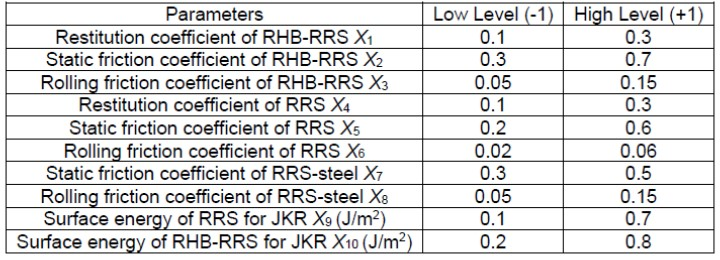Simulation Test

The single sphere model was employed to present the ground RRS particles (Fig. 1a); according to the shape and dimensions, a modified straight four spheres model was used to stand for the RHB particles, in which the coordinates of each of the four spheres were adjusted (Fig. 1b) in this study. The simulation test is shown in Fig. 2.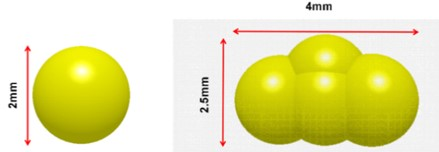(a)                                                        (b)

Fig. 1. Particle model: (a) RRS, (b) RHB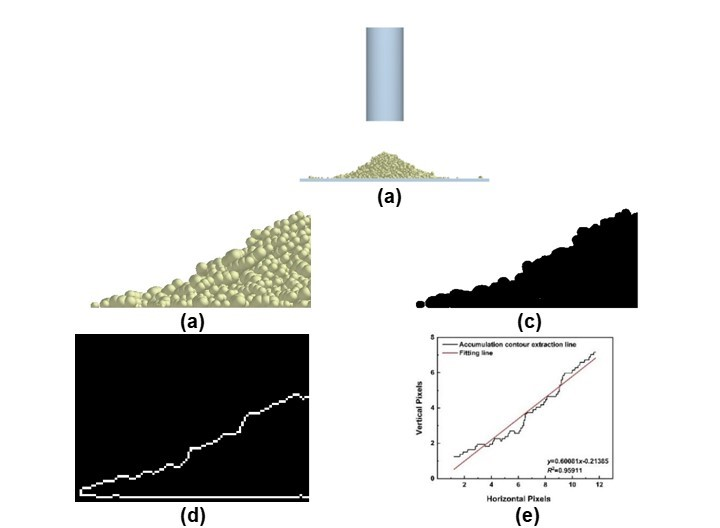Fig. 2. Simulation test of repose angle. (a) The simulated pile of the substrate, (b) original graph, (c) binarization graph, (d) contour extraction curve of the pile and (e) Fitting line

RESULTS AND DISCUSSION

Factor Significance Analysis of the Plackett-Burman Test

The simulation result of the repose angle of the Plackett-Burman test is shown in Table 2, and the significance analysis is given in Table 3. The factors that significantly affected the repose angle were the restitution coefficient of RRS X4, the rolling friction coefficient of RRS X6, the rolling friction coefficient of RRS-steel X8, the surface energy of RRS for JKR X9, and the surface energy RHB-RRS for JKR X10. The other parameters were not highly significant. The rolling friction of RRS-steel was ignored in the regression test because it was imperceptible in the actual test.

Table 2. Design and Results of Plackett-Burman Test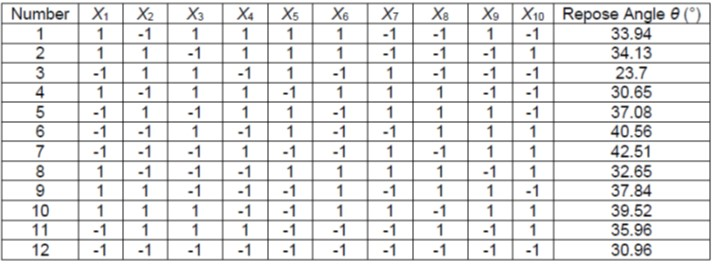Table 3. Significance Analysis of Plackett-Burman Test Parameters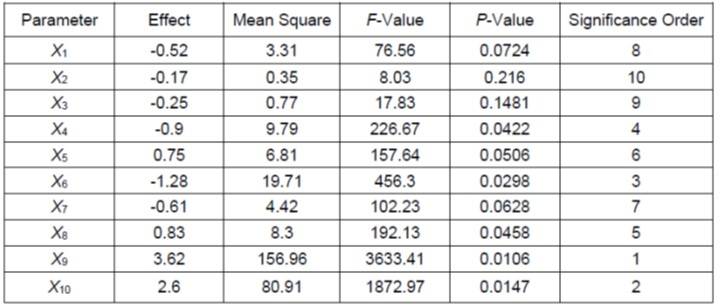Box-Behnken Test and Regression Model

The Box-Behnken experiment of four-factor with three-level was designed to obtain the optimal parameter combination of significant factors (X4, X6, X9, X10) in the simulation test. The repose angle θ was taken as the response value while the other parameters took the intermediate value. A total of 29 experiments were conducted. The coding of test factors is shown in Table 4.

Table 4. Factors and Codes of Box-Behnken Test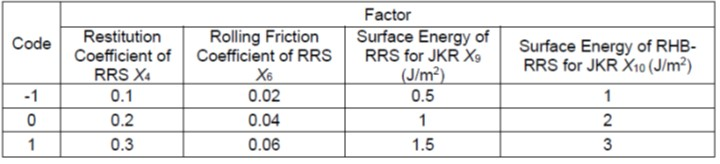Regression analysis

The test protocol and the simulation results are given in Table 5. The multiple regression fitting analysis was performed using Design-Expert (V. 8.0.6, STAS-EASE Inc., Minneapolis, MN, USA), and the regression equation for repose angle was represented by Eq. 2,

θ = 42.50 – 0.37X4 + 1.98X6 + 3.72X9 + 2.55X10 – 0.39X4X6 + 0.11X4X9 – 1.08X4X10 – 2.16X6X9 – 2.20X6X10 – 2.14X9X10 – 0.27X42 – 1.43X62 – 1.60X92 – 3.06X102
(2)

Table 5. Test Plan and Results of Box-Behnken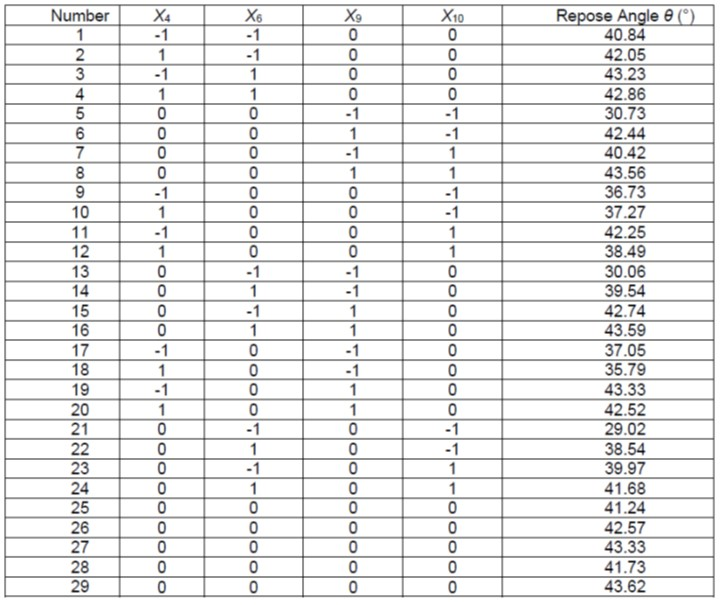The variance analysis results of the experiment are shown in Table 6. It can be observed that the P-value of the regression model was less than 0.01(P < 0.01), the Lack of Fit = 0.0894 was less than 0.05, the determination coefficient R2 was 0.8999, and the adjusted coefficient of determination Radj2 was 0.7998. It can be concluded that the model was well able to express the relationship between the repose angle and the significant factors. The variation coefficient CV= 4.62%, indicated that the test reliability is relatively high. The adequate precision Ap = 11.450, indicated that the model could reasonably predict the substrate repose angle. The significance order of the four factors on the repose angle were X10 > X6 > X9 > X4, and the interactions of X6 and X9, X6 and X10, X9 and X10, X92 had significant effects on the repose angle while the other factors and interactions were not insignificant.

The model was represented as Eq. 3 after eliminating the insignificant items X4, X4X6, X4X9, X4X10, X42, and X62 on the basis of the original model. Variance analysis of regression models is shown in Table 7, and it can be observed that the test accuracy increased to 14.720, indicating that the model can be used to predict the relations between the substrate repose angle and the four significant factors. The optimized quadratic regression equation was developed as Eq. 3:

θ = 41.57 + 1.98X6 + 3.72X9 + 2.55X10 – 2.16X6X9 – 2.20X6X10

– 2.14X9X10 – 1.33X92 – 2.79X102 (3)

Table 6 Variance Analysis of Quadratic Model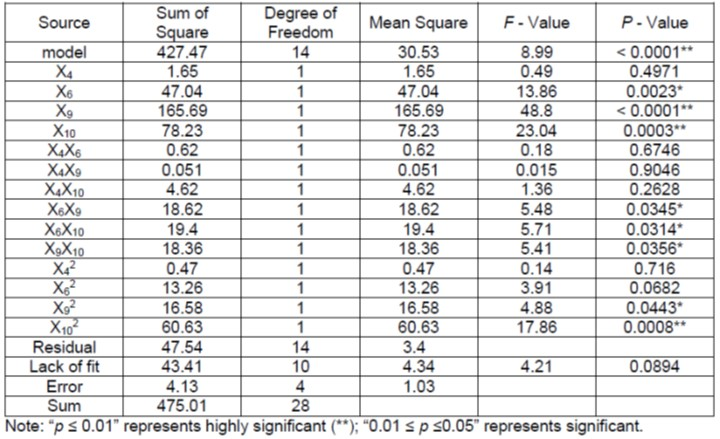Table 7. Variance Analysis of Regression Model Optimization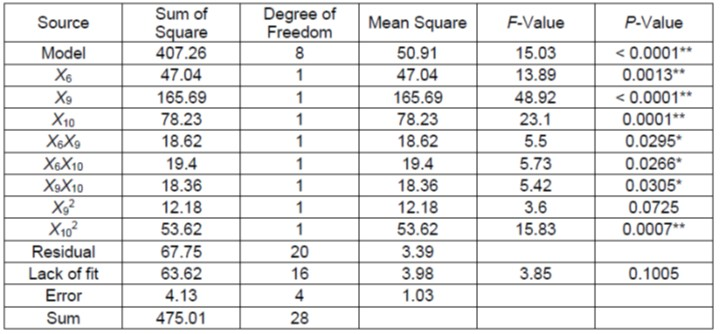Interaction effects analysis

Design-Expert software was employed to draw the 3D response surface of the interaction between the factors. It can be observed from Fig. 3a that the trend of the surface energy of RRS for JKR X9 was slightly steeper than that of rolling friction coefficient X6 of RRS, indicating that the surface energy of RRS for JKR had a more highly significant effect on repose angle. It can be obtained from Fig. 3b that the curve trend of the surface energy of RHB-RRS for JKR X10 gradually became flatter with the increase of the factor value, compared with the curve trend in the direction of rolling friction coefficient of RRS X6, indicating that the surface energy of RHB-RRS for JKR X10 had a more significant impact on the repose angle. According to Fig. 3c, the curve in the direction of the surface energy of RHB-RRS for JKR X10 was similar to that of the surface energy of RRS for JKR X9, indicating that the effects of the two factors on the repose angle are similar.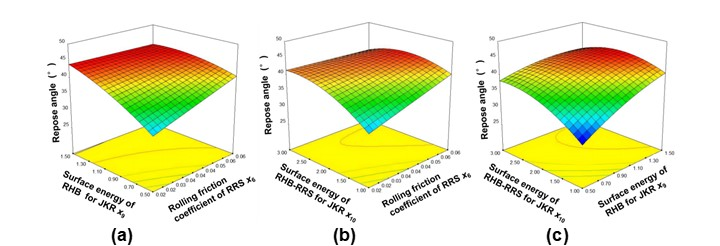Fig. 3. Response surface of significant factors’ interaction. (a) Interaction between X6 and X9, (b) interaction between X6 and X10 and (c) interaction between X9 and X10

Verification Testing

Repose angle verification test

The repose angle, as illustrated in Fig. 4, was tested. To verify the accuracy of the calibration parameters, the real measured repose angle 37.36° was taken as the target value, and the combination of the factors was obtained based on the optimization function of Design-expert. The optimal combination is the restitution coefficient of RRS X4 of 0.20, the rolling friction coefficient of RRS x6 of 0.04, the surface energy of RRS for JKR X9 of 0.53 and the surface energy of RHB-RRS for JKR X10 of 2.11.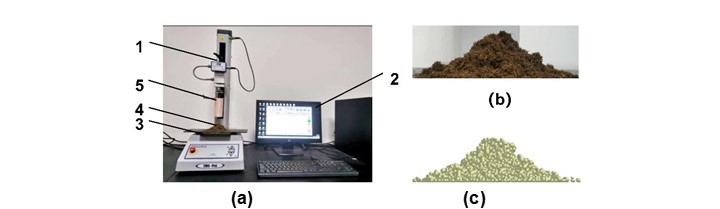Fig. 4. Comparative verification test of repose angle. (a) Real repose angle test: (1) TMS-pro texture analyzer, (2) Automatic control system, (3) Steel plane, (4) Material pile and (5) cylinder; (b) The actual pile of the substrate; (c) The simulated pile of the substrate

The values of X4, X6, X9 and X10 in EDEM were set as the optimal values in the regression model, and the other parameters taking the intermediate level of each factor to obtain the discrete element model of the substrate. The experiment was repeated five times, and the average simulated repose angle was 37.63°. The relative error of repose angles between simulation and experiment values was 0.71 %, indicating that the simulation parameters were reasonable. The results of the verification test and the simulation showed that there were a few local angles exceeded 90°. This might have resulted from the lumps of the substrate materials for the cohesion among them.

Bending strength verification test

To further verify the accuracy of the parameters obtained from the regression model and the discrete element model, the bending strength of the compressed substrate mat of the actual experiment, measured with the electronic universal testing machine, was compared with that of the simulated mat which derived from the EDEM post-processing, as shown in Fig. 5.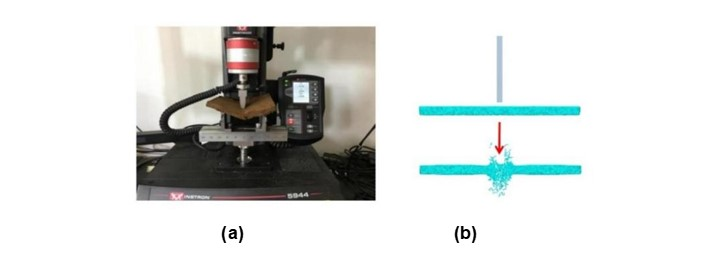Fig. 5. The bending strength verification test. (a) real destruction, (b) simulated destruction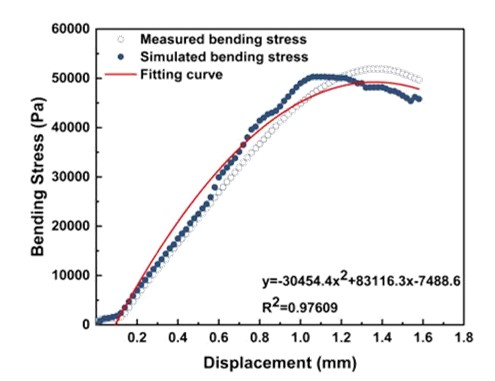Fig. 6. Comparative analysis of bending strength test

The bending stress-displacement curves of the real experiment and simulation test are shown in Fig. 6. The bending strength (the peak of the bending stress curve) obtained from the simulation test was 50,300 Pa, which was close to the measured value of 51,009 Pa. The relative error of the real experimental bending strength was 1.39%, which was larger than that of the accumulation verification (0.71%). The reason is that RHB particles are special-shape particles, and the cohesion will change after compression deformation (Zhang and Shu 2009), resulting in greater error. It can be concluded from Fig. 6 that the determination coefficient R2 of the two curves obtained by fitting was 0.97609, which is close to 1, indicating that the two curves fit well. In conclusion, the discrete element simulation model of gentian substrate block is accurate and reasonable.

CONCLUSIONS

1. The highly significant factors affecting the substrate repose angle were determined through the Placket-Burman method. The restitution coefficient of rotten rice straw (RRS), the rolling friction coefficient of RRS, the surface energy of RRS for the Johnson-Kendall-Roberts (JKR) model, and the surface energy of RHB-RRS for JKR were determined.
2. The optimal discrete parameter combination for repose angle was obtained by Design-Expert as the restitution coefficient of RRS of 0.20, the rolling friction coefficient of RRS of 0.04, the surface energy of RRS for JKR of 0.53, and the surface energy of RHB-RRS for JKR of 2.11.
3. The relative errors of repose angle and bending strength, based on the combination of optimal parameters, between the simulation and the real measurement were 0.71% and 1.39%, respectively, indicating that the simulation parameters of the substrate dispersion element calibration based on the JKR model were reasonable and reliable.

ACKNOWLEDGMENTS

The authors are grateful for the support of the Shenyang Bureau of Science and Technology, Grant No.22-317-2-05; the support of the Educational Department of Liaoning Province, Grant No. LJKMZ20220998；the support of the Ecological Planting and Quality Assurance Project of Authentic Medicinal Materials.

REFERENCES CITED

Bai, S., Yuan, Y., Niu, K., Zhou, L., Zhao, B., Wei, L., Liu, L., Xiong, S., Shi, Z., Ma, Y., et al. (2022). “Simulation parameter calibration and experimental study of a discrete element model of cotton precision seed metering,” Agriculture 12, article 870. DOI: 10.3390/AGRICULTURE12060870.

Chen, X. l., Chi, F. Q., Zhang, J. M., Kuang, E. J., Zhang, S. J., Li, J., Li, W. Q., and Wan, S. M. (2021). “Application of compost maize straw as vegetable grow seedling matrix,” Heilongjiang Agricultural Sciences (1), 43-46. DOI: 10. 11942/j.issn1002-2767.2021.1.0043. (in Chinese)

Fan, J., Li, Y., Wang, B., Gu, F., Wu, F., Yang, H., Yu, Z., and Hu, Z. (2022). “An experimental study of axial Poisson’s ratio and axial Young’s modulus determination of potato stems using image processing,” Agriculture 12, 1026. DOI: 10.3390/AGRICULTURE12071026.

Guo, J. L., Zhi, L. H., and Zhang, G. (2011). “Effect of substrate of corn stalks on growth of soilless culture of lettuce,” Northern Horticulture (13), 34-35. (in Chinese)

Li, J. W., Tong, J., Hu, B., Wang, H. B., Mao, C. Y., and Ma, Y. H. (2019). “Calibration of parameters of interaction between clayey black soil with different moisture content and soil-engaging component in northeast China,” Transactions of the Chinese Society of Agricultural Engineering (Transactions of the CSAE) 35(6), 130-140. DOI: 10.11975/j.issn.1002-6819.2019.06.016. (in Chinese)

Li, Y. K., Sun, Y. Z., and Bai, X. W. (2015). “Extrusion process of corn stalk powder in single orifice die processing based on discrete element method,” Transactions of the Chinese Society of Agricultural Engineering (Transactions of the CSAE) 31(20), 212-217. DOI: 10.11975/j.issn.1002-6819.2015.20.030. (in Chinese)

Liu, A. M., Xiang, Y. C., Tian, D. K., and Mo, H. B. (2015). “Effects of biochar on plant growth and uptake of heavy mental cadmium,” Journal of Soil and Water Conservation 27(5). DOI: 10.13870/j.cnki.stbcxb.2013.05.002. (in Chinese)

Mi, G., Liu, Y., Wang, T., Dong, J., Zhang, S., Li, Q., Chen, K., and Huang, Y. (2022). “Measurement of physical properties of sorghum seeds and calibration of discrete element modeling parameters,” Agriculture 12, article 681. DOI: 10.3390/AGRICULTURE12050681

Mohammadreza, A., Maryam, A., Mojtaba, G. A., and Bayly, A. H. (2018). “A methodology for calibration of DEM input parameters in simulation of segregation of powder mixtures, a special focus on adhesion,” Powder Technology 339, 789-800. DOI: 10.1016/j.powtec.2018.08.028.

Na, R. S., Li, S., Li, X., Jia, K. F., and Zhang, W. G. (2022). “Discrete element simulation on dense forming characteristics for corn straw mixture pellet feed”, Forging & Stamping Technology 47(4), 162-169. DOI: 10.13330/j.issn.1000-3940.2022.04.022 (in Chinese)

Qin, T. (2021). “Application of eggplant straw composite substrate in balsam pear seedling,” Journal of Henan Agricultural Sciences 50(5), 115-121. DOI: 10.15933/j.cnki.1004-3268.2021.05.016 (in Chinese)

Qiu, Y., Guo, Z., Jin, X., Zhang, P., Si, S., and Guo, F. (2022). “Calibration and verification test of cinnamon soil simulation parameters based on discrete element method,” Agriculture 12, article 1082. DOI: 10.3390/AGRICULTURE12081082

Rodrigues, C., Karmali, A., and Machado, J. (2019). “The extracts of Gentiana lutea with potential cytotoxic effects on human carcinoma cell lines: A preliminary study,” European Journal of Integrative Medicine 27, 34-38. DOI: 10.1016/j.eujim.2019.02.008

Saha, A., Basak, B. B., Gajbhiye, N. A., Kalariya, K. A., and Manivel, P. (2019). “Sustainable fertilization through co-application of biochar and chemical fertilizers improves yield, quality of Andrographis paniculata and soil health,” Industrial Crops and Products 140(C), 111607-111607. DOI: 10.1016/j.indcrop.2019.111607

Scortichini, M., and Rossi, M. P. (1991). “Preliminary in vitro evaluation of the antimicrobial activity of terpenes and terpenoids towards Erwinia amylovora,” Journal of Applied Bacteriology 71(2), 109-112. DOI: 10.1111/j.1365-2672.1991.tb02963.x

Tang, H. M. (2019). “Effects of microbial agents and biochar on the yield and quality of Pinellia ternata and soil microecology”, Master’s thesis, Huazhong Agricultural University, Wuhan, China. DOI: 10.27158/d.cnki.ghznu.2019.000724. (in Chinese)

Tian, X., L., Cong, X., Qi, J., T., Guo, H., Li, M. and Fan, X., H. (2021). “Parameter calibration of discrete element model for corn Straw-soil mixture in black soil areas,” Transactions of the Chinese Society for Agricultural Machinery 52(10). DOI: 10.6041/j.issn.1000-1298.2021.10.010. (in Chinese)

Wang, W. W., Cai, D. Y., Xie, J. J., Zhang, C. L., Liu, L. C., and Chen, L. Q. (2021). “Parameters calibration of discrete element model for corn stalk powder compression simulation,” Transactions of the Chinese Society for Agricultural Machinery 52(3), 127-134. DOI: 10.6041/j.issn.1000-1298.2021.03.013. (in Chinese)

Wang, Y., H., and Hou, J. W. (2010). “Application of well-composted corn-stover substrate to China-aster (Callistephus chinensis) seeding,” Journal of Anhui Agri Sci 38(29), 16175-16176. DOI: 10.13989/j.cnki.0517-6611.2010.29.126. (in Chinese)

Xin, M. J., Chen T. Y., Zhang Q., Jiao, J. K., Bai, X. W., Song, Y. Q., Zhao, R., and Wei, C. (2017). “Parameters optimization for molding of vegetable seedling substrate nursery block with rice straw,” Transactions of the Chinese Society of Agricultural Engineering 33 (16), 219-255. DOI: 10.11975/j.issn.1002-6819.2017.16.029. (in Chinese)

Yang, L., Wen, Z. W., Fu, J., Xia, R., Pan, G. X., and Yang, L. M. (2020). “Effects of biochar on seedling and soil quality of continuous cropping Panax ginseng,” Journal of Chinese Medicinal Materials 43(4), 791-796. DOI: 10.13863/j.issn1001-4454.2020.04.002. (in Chinese)

Zhang, C., and Shu, G. P. (2009). “Effect of particle shape on biaxial tests simulated by particle flow code,” Chinese Journal of Geotechnical Engineering 31(08), 1281-1286. (in Chinese)

Zhang, C. G., Zhou, L. G., Wu, M. G., and Fu, M. J. (2010). “Screening of the optimum formula for Radix gentianae,” Journal of Anhui Agricultural Sciences 38(14), 7331-7333. DOI: 10.13989/j.cnki.0517-6611.2010.14.095. (in Chinese)

Article submitted: October 5, 2022; Peer review completed: December 31, 2022; Revised version received and accepted: January 19, 2023; Published: January 25, 2023.

DOI: 10.15376/biores.18.1.1999-2010